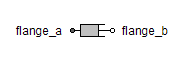Voltage Controller - MapleSim Help

Voltage Controller

Voltage controllerDescription The Voltage Controller component is a simple voltage-controller. The desired rms values of the d- and q-components of the space phasor current in rotor fixed coordinate system are given by inputs ${i}_{d,\mathrm{rms}}$ and ${i}_{q,\mathrm{rms}}$. Using the given rotor position (input $\mathrm{\phi }$), the actual three-phase currents are measured and transformed to the d-q coordinate system. Two PI-controllers determine the necessary d- and q- voltages, which are transformed back to three-phase (output $y$). They can be used to feed a voltage source which in turn feeds a permanent magnet synchronous machine. Note: No care is taken for current or voltage limiting, as well as for field weakening.Connections

 Name Description Modelica ID $y$ Three-phase real output y ${i}_{d,\mathrm{rms}}$ Real input; d-component of desired rms current id_rms ${i}_{q,\mathrm{rms}}$ Real input; q-component of desired rms current iq_rms $\mathrm{\phi }$ Real input; rotor position in radians phi ${i}_{\mathrm{actual}}$ Three-phase real input; actual rms current iActualParameters

 Name Default Units Description Modelica ID $p$ Number of pole pairs p ${f}_{s,\mathrm{nom}}$ $\mathrm{Hz}$ Nominal frequency fsNominal ${V}_{s,\mathrm{oc}}$ $V$ Open circuit RMS voltage per phase at_f[s,nom] VsOpenCircuit ${R}_{s}$ $\mathrm{\Omega }$ Stator resistance per phase Rs ${L}_{d}$ $H$ Inductance in d-axis Ld ${L}_{q}$ $H$ Inductance in q-axis Lq Decoupling $\mathrm{false}$ True means use decoupling network decouplingModelica Standard Library The component described in this topic is from the Modelica Standard Library. To view the original documentation, which includes author and copyright information, click here.